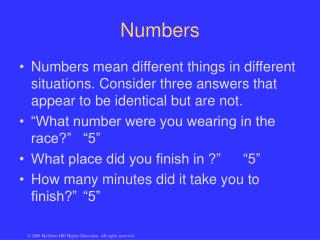DownloadDownload PresentationNumbers

# Numbers

Télécharger la présentation## Numbers

- - - - - - - - - - - - - - - - - - - - - - - - - - - E N D - - - - - - - - - - - - - - - - - - - - - - - - - - -
##### Presentation Transcript

1. Numbers • Numbers mean different things in different situations. Consider three answers that appear to be identical but are not. • “What number were you wearing in the race?” “5” • What place did you finish in ?” “5” • How many minutes did it take you to finish?” “5”

2. Number Scales • Nominal Scale • Ordinal Scale • Interval • Ratio

3. Nominal Scale: This scale refers to a classificatory approach, i.e., categorizing observations. Distinct characteristics must exist to categorize: gender, race essentially you can only be assigned one group. KEY: to distinguish one from another.

4. Ordinal Scale: This scale puts order into categories. It only ranks categories by ability, but there is no specific quantification between categories. It is only placement, e.g., judging a swimming race without a stopwatch, i.e., there is no quantitiy to determine the difference between ranks. KEY: placement without quantification.

5. Interval Scale: This scale adds equal intervals between observed categories. We know that 75 points is halfway between scores of 70 and 80 points on a scale. KEY: how much was the difference between 1st and 2nd place?

6. Ratio Scale: this scale has all the qualities of an interval scale with the added property of a true zero. Not all qualities can be assigned to a ratio scale. KEY: quality of measurement must represent a true zero.

7. Normal Distribution • Most statistical methods are based on assumption that a distribution of scores is normal and that the distribution can be graphically represented by the normal curve (bell-shaped). • Normal distribution is theoretical and is based on the assumption that the distribution contains an infinite number of scores.

8. Characteristics of Normal Curve • Bell-shaped curve • Symmetrical distribution about vertical axis of curve • Greatest number of scores found in middle of curve • All measures of central tendency at vertical axis

9. mean median mode

10. Measures of Central Tendency • descriptive statistics • describe the middle characteristics of the data (distribution of scores); represent scores in a distribution around which other scores seem to center • most widely used statistics • mean, median, and mode

11. Mean The arithmetic average of a distribution of scores; most generally used measure of central tendency. Characteristics • Most sensitive of all measures of central tendency • Most appropriate measure of central tendency to use for ratio data (may be used on interval data) • Considers all information about the data and is used to perform other statistical calculations • Influenced by extreme scores, especially if the distribution is small

12. Median Score that represents the exact middle of the distribution; the fiftieth percentile; the score that 50% of the scores are above and 50% of the scores are below. Characteristics • Not affected by extreme scores. • A measure of position. • Not used for additional statistical calculations. • Represented by Mdn or P50.

13. Mode Score that occurs most frequently; may have more than one mode. Characteristics Least used measure of central tendency. Not used for additional statistics. Not affected by extreme scores.

14. Which Measure of Central Tendency is Best for Interpretation of Test Results? • Mean, median, and mode are the same for a normal distribution, but often will not have a normal curve. • The farther away from the mean and median the mode is, the less normal the distribution. • The mean and median are both useful measures. • In most testing, the mean is the most reliable and useful measure of central tendency; it is also used in many other statistical procedures.

15. Measures of Variability • To provide a more meaningful interpretation of data, you need to know how the scores spread. • Variability - the spread, or scatter, of scores; terms dispersion and deviation often used • With the measures of variability, you can determine the amount that the scores spread, or deviate, from the measures of central tendency. • Descriptive statistics; reported with measures of central tendency

16. Range Determined by subtracting the lowest score from the highest score; represents on the extreme scores. Characteristics 1. Dependent on the two extreme scores. 2. Least useful measure of variability. Formula: R = Hx - Lx

17. Standard Deviation • Most useful and sophisticated measure of variability. • Describes the scatter of scores around the mean. • Is a more stable measure of variability than the range or quartile deviation because it depends on the weight of each score in the distribution. • Lowercase Greek letter sigma is used to indicate the the standard deviation of a population; letter s is used to indicate the standard deviation of a sample. • Since you generally will be working with small samples, the formula for determining the standard deviation will include (N - 1) rather than N.

18. Characteristics of Standard Deviation 1. Is the square root of the variance, which is the average of the squared deviations from the mean. Population variance is represented as F2 and the sample variance is represented as s2. 2. Is applicable to interval and ratio data, includes all scores, and is the most reliable measure of variability. 3. Is used with the mean. In a normal distribution, one standard deviation added to the mean and one standard deviation subtracted from the mean includes the middle 68.26% of the scores.